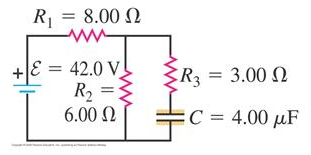# Problem: The capacitor in the figure shown is initially uncharged. The switch is closed at t = 0.What is the final charge on the capacitor?

###### FREE Expert Solution

Voltage divider rule:

The charge stored on a capacitor:

$\overline{){\mathbf{Q}}{\mathbf{=}}{\mathbf{C}}{\mathbf{V}}}$

When the capacitor is fully charged, there is no current through R3. The voltage across the capacitor is equal to the voltage across R2

VC = V2 = ε[R2/(R1 + R2)] = 42.0[6.00/(6.00 + 8.00)] = 18 V

98% (344 ratings)###### Problem Details

The capacitor in the figure shown is initially uncharged. The switch is closed at t = 0.

What is the final charge on the capacitor?